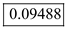# Two identical 5-ft-long rods connected by a pin at B are placed between two walls and a horizontal suri ace as shown. Denoting by µs, the coefficient of static friction at A, B, and C determine the smallest value of µs for which equilibrium is maintained.

Question-AnswerCategory: Engineering MechanicsTwo identical 5-ft-long rods connected by a pin at B are placed between two walls and a horizontal suri ace as shown. Denoting by µs, the coefficient of static friction at A, B, and C determine the smallest value of µs for which equilibrium is maintained.

Two identical 5-ft-long rods connected by a pin at B are placed between two walls and a horizontal suri ace as shown. Denoting by µs, the coefficient of static friction at A, B, and C determine the smallest value of µs for which equilibrium is maintained.Step: 1

Draw the free body diagram of the rod AB: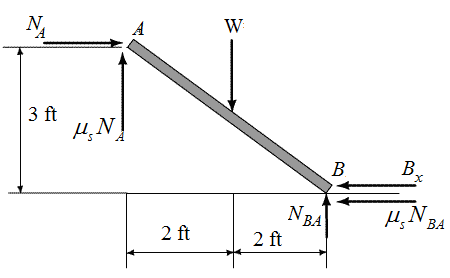Step: 2

Let
The normal force at point A isThe frictional force at point A is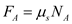The normal force at point B isThe frictional force at point B is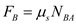The length of the each rod is l
At pin B, the degree of freedom is one as it having motion along the x-direction. Hence, at pin B have only x-component force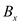and y-component force is zero
Assume that the moment in the anticlockwise direction is positive.…… (1)

Step: 3

Take the equilibrium forces along y-direction:…… (2)
Take the equilibrium forces along x-direction:…… (3)

Step: 4

Draw the free body diagram of rod BC: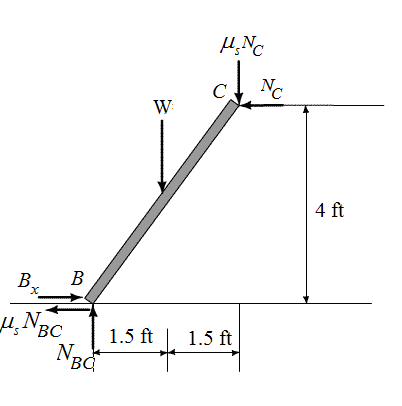Step: 5

Let
The normal force at point C isThe frictional force at point A isThe normal force at point B isThe frictional force at point B is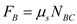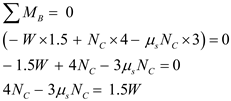…… (4)

Take the equilibrium forces along y-direction:…… (5)
Take the equilibrium forces along x-direction:…… (6)

Step: 6

Substitute the equation (3) in equation (6)Substitute the values of NAB and NBC from equation (2) and equation (5)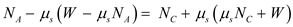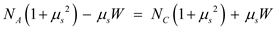…… (7)
Substitute the values of NA and NC from equation (1) and equation (4) in equation (7)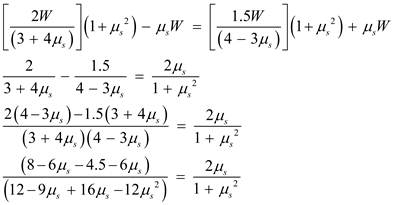Step: 7

Simplify and solve the above equation for the value of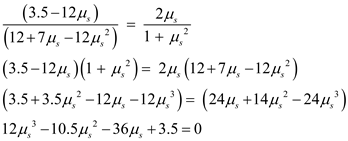From the above equation solve for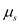Therefore, the smallest value offor which equilibrium is maintained is